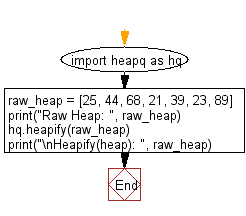﻿ Python heap queue algorithm: Accepts an arbitrary list and converts it to a heap using Heap queue algorithm - w3resource

# Python: Accepts an arbitrary list and converts it to a heap using Heap queue algorithm

## Python heap queue algorithm: Exercise-4 with Solution

Write a Python function that accepts an arbitrary list and converts it to a heap using the heap queue algorithm.

Sample Solution:

Python Code:

``````import heapq as hq
raw_heap = [25, 44, 68, 21, 39, 23, 89]
print("Raw Heap: ", raw_heap)
hq.heapify(raw_heap)
print("\nHeapify(heap): ", raw_heap)
```
```

Sample Output:

```Raw Heap:  [25, 44, 68, 21, 39, 23, 89]

Heapify(heap):  [21, 25, 23, 44, 39, 68, 89]
```

Flowchart:Python Code Editor:

Have another way to solve this solution? Contribute your code (and comments) through Disqus.

What is the difficulty level of this exercise?

Test your Programming skills with w3resource's quiz.

﻿